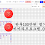## Thursday, December 10, 2020

### Some Loxodromes

Loxodromes are generated by curves of constant bearing on a sphere (always making a constant angle with circles of latitude). If you go in a direction and proceed forever in something that's NOT one of the 4 cardinal directions, you'll get something very interesting. It's not really obvious that you actually will spiral forever around a pole. These have the simplest expression in isothermal coordinates we talked about in the last post: they can be represented simply as straight lines in the $uv$ plane (well, if you allow $v$ to range over all of $\mathbb R$ and wrap around; if you insist on $v$ only being in an interval of length $2\pi$, then after the line goes off the top edge, you make it jump back to the bottom edge so you get something that looks like this: $/////$. So the full parametrization would be: $(\operatorname{sech}u \cos(au+b), \operatorname{sech}u \sin(au+b), \tanh u)$. It is easy to intuitively see why the angle between these curves and the $\theta$ curves are constant: the $uv$-plane is first mapped (conformally) to the whole $z$-plane by $(e^u\cos v, e^u\sin v)$, and then the (conformal) stereographic projection wraps it all up. The same cannot be said of the $\varphi\theta$ plane (to confirm, of course, you need to mess with metric coefficients in that last post).

1.2.